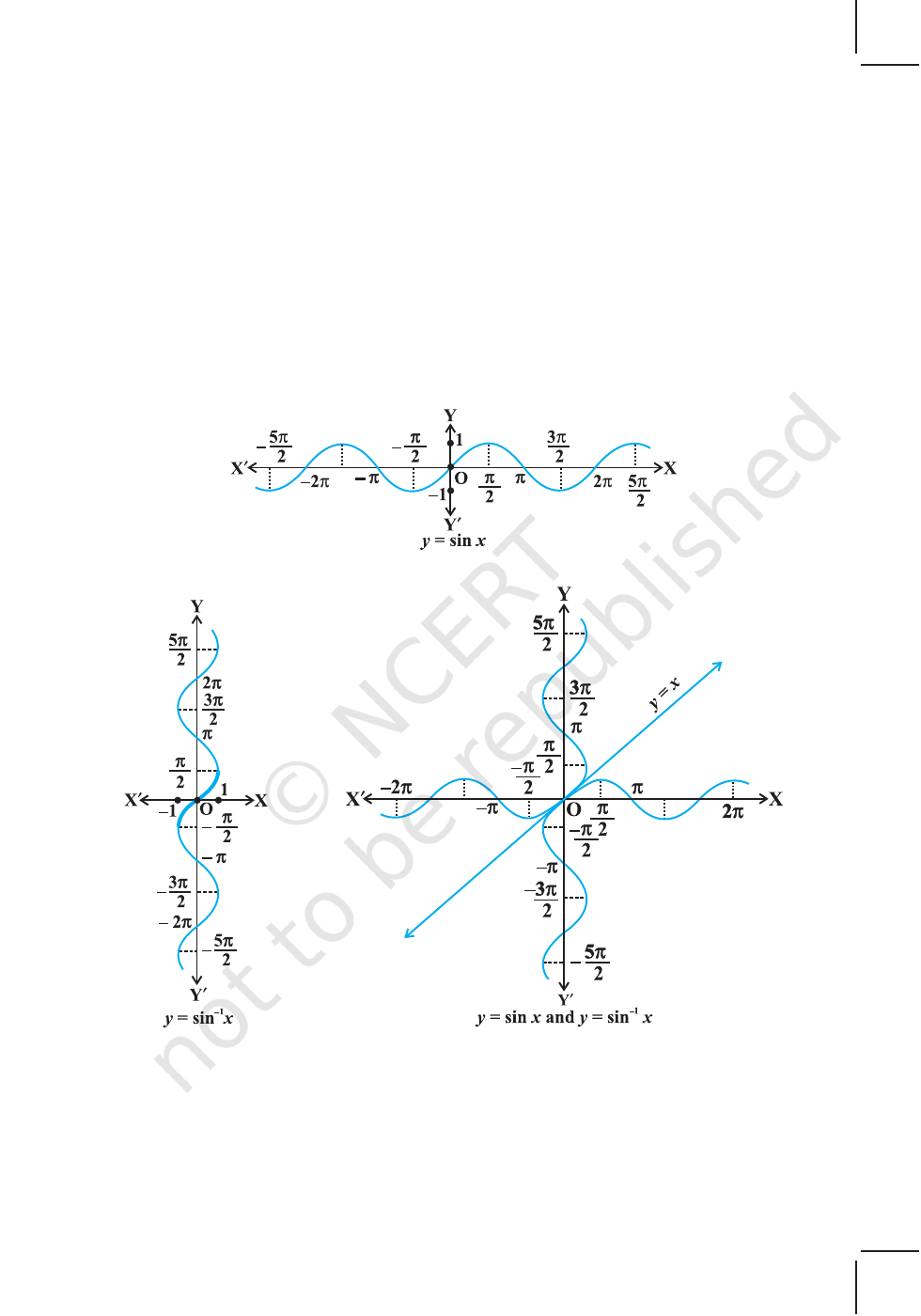v
Mathematics, in general, is fundamentally the science of
self-evident things. — FELIX KLEIN
v
2.1 Introduction
In Chapter 1, we have studied that the inverse of a function
f, denoted by f
–1
, exists if f is one-one and onto. There are
many functions which are not one-one, onto or both and
hence we can not talk of their inverses. In Class XI, we
studied that trigonometric functions are not one-one and
onto over their natural domains and ranges and hence their
inverses do not exist. In this chapter, we shall study about
the restrictions on domains and ranges of trigonometric
functions which ensure the existence of their inverses and
observe their behaviour through graphical representations.
Besides, some elementary properties will also be discussed.
The inverse trigonometric functions play an important
role in calculus for they serve to define many integrals.
The concepts of inverse trigonometric functions is also used in science and engineering.
2.2 Basic Concepts
In Class XI, we have studied trigonometric functions, which are defined as follows:
sine function, i.e., sine : R [– 1, 1]
cosine function, i.e., cos : R [– 1, 1]
tangent function, i.e., tan : R – { x : x = (2n + 1)
2
π
, n Z} R
cotangent function, i.e., cot : R – { x : x = nπ, n Z} R
secant function, i.e., sec : R – { x : x = (2n + 1)
2
π
, n Z} R – (– 1, 1)
cosecant function, i.e., cosec : R – { x : x = nπ, n Z} R – (– 1, 1)
Chapter
2
INVERSE TRIGONOMETRIC
FUNCTIONS
Aryabhata
(476-550 A. D.)
2019-2034 MATHEMATICS
We have also learnt in Chapter 1 that if f : XY such that f(x) = y is one-one and
onto, then we can define a unique function g : YX such that g(y) = x, where
x X
and y = f(x), y
Y. Here, the domain of g = range of f and the range of g = domain
of f. The function g is called the inverse of f and is denoted by f
–1
. Further, g is also
one-one and onto and inverse of g is f. Thus, g
–1
= (f
–1
)
–1
= f. We also have
(f
–1
o f ) (x) = f
–1
(f (x)) =
f
–1
(y) = x
and (f o f
–1
) (y) = f (f
–1
(y))
= f(x) = y
Since the domain of sine function is the set of all real numbers and range is the
closed interval [–1, 1]. If we restrict its domain to
,
2 2
π π
, then it becomes one-one
and onto with range [– 1, 1]. Actually, sine function restricted to any of the intervals
3
2 2
π π
,
,
,
2 2
,
3
,
2 2
π π
etc., is one-one and its range is [–1, 1]. We can,
therefore, define the inverse of sine function in each of these intervals. We denote the
inverse of sine function by sin
–1
(arc sine function). Thus, sin
–1
is a function whose
domain is [– 1, 1] and range could be any of the intervals
3
,
2 2
π π
,
,
2 2
or
3
,
2 2
π π
, and so on. Corresponding to each such interval, we get a branch of the
function sin
–1
. The branch with range
,
2 2
−π π
is called the principal value branch,
whereas other intervals as range give different branches of sin
–1
. When we refer
to the function sin
–1
, we take it as the function whose domain is [–1, 1] and range is
,
2 2
−π π
. We write sin
–1
: [–1, 1]
,
2 2
π π
From the definition of the inverse functions, it follows that sin (sin
–1
x) = x
if – 1 x 1 and sin
–1
(sin x) = x if
2 2
x
π π
. In other words, if y = sin
–1
x, then
sin y = x.
Remarks
(i) We know from Chapter 1, that if y = f(x) is an invertible function, then x = f
–1
(y).
Thus, the graph of sin
–1
function can be obtained from the graph of original
function by interchanging x and y axes, i.e., if (a, b) is a point on the graph of
sine function, then (b, a) becomes the corresponding point on the graph of inverse
2019-20INVERSE TRIGONOMETRIC FUNCTIONS 35
of sine function. Thus, the graph of the function y = sin
–1
x can be obtained from
the graph of y = sin x by interchanging x and y axes. The graphs of y = sin x and
y = sin
–1
x are as given in Fig 2.1 (i), (ii), (iii). The dark portion of the graph of
y = sin
–1
x represent the principal value branch.
(ii) It can be shown that the graph of an inverse function can be obtained from the
corresponding graph of original function as a mirror image (i.e., reflection) along
the line y = x. This can be visualised by looking the graphs of y = sin x and
y = sin
–1
x as given in the same axes (Fig 2.1 (iii)).
Like sine function, the cosine function is a function whose domain is the set of all
real numbers and range is the set [–1, 1]. If we restrict the domain of cosine function
to [0, π], then it becomes one-one and onto with range [–1, 1]. Actually, cosine function
Fig 2.1 (ii)
Fig 2.1 (iii)
Fig 2.1 (i)
2019-20